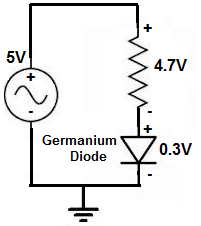﻿ What is the Voltage Drop Across a Germanium Diode

# Quick Q&AQuestion: What is the Voltage Drop Across a Germanium Diode?

A Germanium Diode has a voltage drop of approximately 0.3V across its leads.

A diode is a device that drops a specific voltage across its terminals. It is necessary that a diode get this voltage so that the diode can conduct. Without this voltage, the diode would not meet its threshold voltage needed to conduct current, and the circuit could not have current flow through it.

Below is an example of a circuit containing a germanium diode:You can see that the Germanium diode drops 0.3V across its terminals. Therefore, the resistor or load of the circuit gets the remaining supply voltage, which is 4.7V.

The other kind of diode is a silicon diode. A silicon diode drops approximately 0.7V across its terminals. Therefore, a germanium diode uses less voltage than a silicon one.